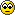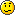# IP Classless

Hello, I am new to this group and have a question for the experts.

Suppose I have a network 192.168.100.0/28.
What would be the first USEABLE subnet assuming "no ip classless".
Also, what would be the first USEABLE address of the first useable subnet.

I have searched for some decent information regarding "no ip classless" and have come up empty.

I thought that 192.168.100.1 would be the first useable address of the first subnet (subnet zero) and 192.168.100.17 would be the first useable address for the second subnet. Can someone guide me in the right direction?

Thanks,
B

• There's more to assume than just IP classless, (ip zubnet-zero must be issued and complete network must support CIDR)

We'll have our own Subnetting TechNote online soon, but you might want to check out this site: www.learntosubnet.com

I hope this helps, otherwise let me know...
• Tango Tango do you know the answer to this problem. Cos when i work it out i am getting the answers:
192.168.16.0 as the first useable subnet.
192.168.16.1 as the first useable address in the first usable subnet.
I could be wrong cos i am new to subnetting too. So i would appreciate it very much if you had the correct answer to see if i am on the right track to.
If you dont it would be nice if one of the experts could give the correct answer like Webmaster.
hint hint.• Try this one:

ftp://ftp.3com.com/pub/utilbin/win32/3CIPCalc.zip• Ok thats wrong. I calculated it as if it was a class B address.
One question webmaster, in the case of this address you are using 4 bits from the Host portion to create subnets and 4 bits for hosts. i thought using all 0's in the subnet portion was not a valid address.
According to the calculator the first host address is: 192.168.100.1
According to the theory i have been taught you can't use the subnet portion as all 0's or all 1's so that would make the first host address: 192.168.100.17 Is this theory correct or does this theory only apply to the host portion of an IP address?
• Ok, here's how I'd do it

192.168.100.0/28 = 192.168.100.0 with subnet mask 255.255.255.240

sm = 11111111.11111111.11111111.11110000

4 bits borrowed
Our rule was that the value of the last bit borrowed was the first subnet
The last bit borrowed is 16 (the 1 to the left of 0000)

so the first network is 192.168.100.16

To calculate no. of hosts on subnet, 2^n - 2, where n=no. of host bits.
n=4, hosts are 14 per subnet so
the second network is 192.168.100.32
and 192.168.100.48, 192.168.100.64, 192.168.100.80, 192.168.100.96 etc

In the first network 192.168.100.16 is the network ID, 192.168.100.17 is the first usable address, 192.168.100.30 is the last usable address and192.168.100.31 is the broadcast address.

if u use VLSM then 192.168.100.0 is ur first subnet and 192.168.100.1 is the first address and the the router needs to be configured as IP subnet zero. Exactly what that means .... I dunno .. but it's using all the subnets, none go to waste.

About the 172 subnet, it's class B and ur teacher is right. Default subnet mask is 255.255.0.0. U r borrowing 4 bits
The first subnet is 172.16.16.0
The second subnet is 172.16.32.0
There are a lot of hosts on each subnet, 2^12 - 2 (there are 12 host bits)
2048-2= 2046 hosts on each subnet. Your answer would give 254 hosts only.

Roger
Yahoo Instant Messanger : spacemancw
• Thanks spacemancw
So it is safe to say that if you have a class B address with 4 bits borrowed for subnetting you cannot use all 0's or all 1's even in the subnet portion.
With an network address of 172.16.0.0 and a subnet mask of 255.255.240.0.
Your first usable host on the first usable subnet would start from:
N.N.00010000.00000001 which would = 172.16.16.1.

N.N.00000000.00000001 = 172.16.0.1 would be the wrong answer.

If this is the case, webmaster i dont think its a good idea to recommend that caculator.
cos it says that 172.16.0.1 is the first usable host.• > N.N.00000000.00000001 = 172.16.0.1 would be the wrong answer.

Again .. if u were using subnet zero u could use 172.16.0.0 as the first network and so 172.16.0.1

But I think in your CCNA class subnet zero is not covered and they stick with the hardcore formula. So on paper 172.16.16.0 is the first network but VLSM allows 172.16.0.0 to be the first network. If I am wrong about that I would like someone to let me know.
So for ur class stick with the original formulas.

Roger
Yahoo Instant Messanger : spacemancw
• Please note that subnet zero has no relation with VLSM....

It is CIDR that allows subnet zero and all 1s broadcast...

On Cisco exams you will have to use both formulas, but they will be clear on when to do so... they'll mention CIDR or subnet zero.
• Thanks guys you cleared up a lot for me. Being new to subnetting its easy to get confused. Maybe i jumpped to far ahead of my self. But i will keep that tip for final exam webmaster:
[It is CIDR that allows subnet zero and all 1s broadcast...

On Cisco exams you will have to use both formulas, but they will be clear on when to do so... they'll mention CIDR or subnet zero.
]
Thanks